### Home > CALC > Chapter 3 > Lesson 3.2.1 > Problem3-47

3-47.
1. After completing the Ramp Lab in Chapter 2, Marquita decided to ride her bicycle down a nearby hill to gain more data on rolling objects. The data she collected is shown in the table below. Homework Help ✎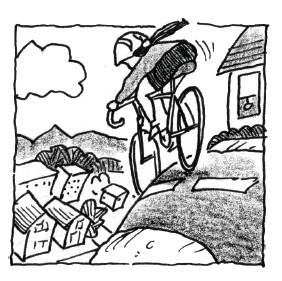Time (sec) Distance (m) 0 2 3.1 6 7.5 9 0 3.8 9.1 32.7 49.7 69.5

1. Can Marquita use this table to determine her exact velocity at t = 6. Explain.

2. Approximate Marquita's velocity at t = 6 using Hana' s method.

3. Marquita' s teacher observed that her data fits the function d(t) = 0.789t2 + 0.703t − 0.338. Assuming that her teacher is correct, compute Marquita's exact velocity at t = 6 and t = 10.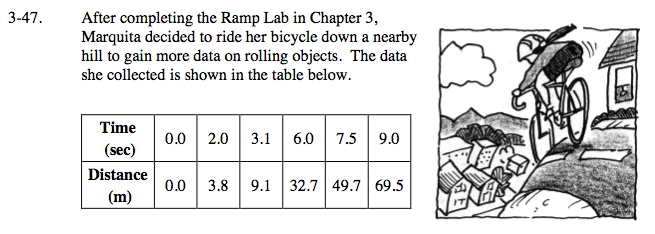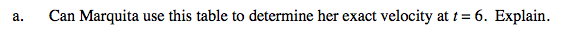Tables only give us select values of a function. What values are we given that are close to t = 6? Are these values close enough to find Marquita's exact velocity at t = 6?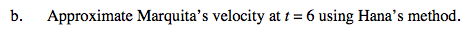Note: Since we do note know values that are infinitely close to t = 6, we can find Marquita's AROC (average velocity), not her IROC (actual velocity).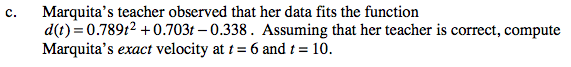Use the Power Rule to find the IROC/derivative/slope function/velocity funciton.Then evaluate at t = 6 and again at t = 10.